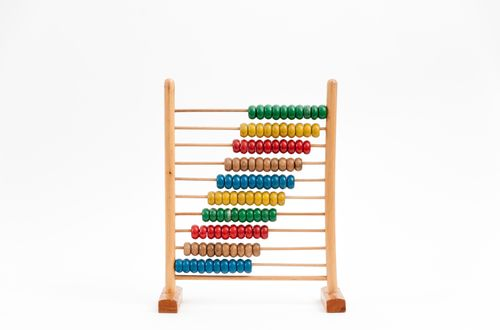# How to simplify ratios

Want to find out how to simplify ratios? We've provided a step-by-step guide of how to simplify ratios to teach you everything you need to know.## Step 1: Find the greatest common factor

Look for the greatest common factor (GCF) shared by the values of the ratios. If we want to simplify 56:70, we’ll need to determine the value of its GCF.

## Step 2: Divide by the greatest common factor

Divide all the values by the GCF. So, for our example, we can divide 56 and 70 by 14.

## Step 3: Rewrite with simplified values

Once the values are simplified, rewrite the ratio with the simplified values. This means that:

## Examples of how to simplify ratios

Q1) Simplify the following ratios. a. 80:90 b. 64:144 c. 128:160

Determine the greatest common factor shared by the values found on each of the ratios. 80=10×8 64=16×4 128=32×4 90=10×9 144=16×9 160=32×5

Once we have the GCF shared by the values on each ratio, we divide the values by the GCF.

80÷10=8 64÷16=4 128÷32=4 90÷10=9 144÷16=9 160÷32=5

Write the ratios with their simplified ratios.

a. 80:90=8:9 b. 64:144=4:9 c. 128:160=4:5

Q2) Jenny allots \$1430 for her monthly utility bills and \$4400 for her monthly rent. What is the ratio of the amount that she spends on monthly utility bills to her monthly rent?

We want to find the simplified ratio of \$1430 for utility bills and \$4400 for monthly bills.

1430:4400

First, let’s determine the greatest common factor (GCF) shared by the values.

1430=110×13 4400=110×40

Divide both values by 110 to find the simplified form of the ratio.

1430÷110=13 4400÷110=40

So, rewriting the ratio, we have 1430:4400=13:40

This means that the ratio that Jenny pays for her utility bills to the amount she spends on rent is 13:40.

#### Join today

The fastest way to practice

Unlock our complete testing platform and improve faster that ever.